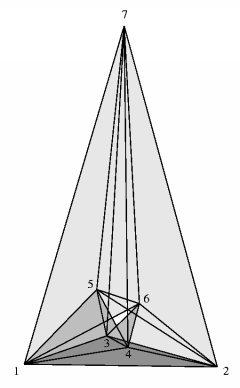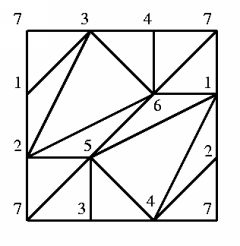Császár's TorusElectronic Geometry Model No. 2001.02.069

Frank H. Lutz

Description

Császár's torus  is one of 72 distinct polyhedral realizations  of Möbius' unique vertex-minimal triangulation of the torus with seven vertices .

Every triangulation of a surface M with Euler characteristic chi(M) has at least n >= (7+sqrt(49-24 chi(M)))/2 vertices, and, with few exceptions, this bound is sharp (, ). If equality holds, then the triangulation is neighborly, i.e., every pair of vertices is connected by an edge. In particular, neighborly triangulations of orientable surfaces can only occur for surfaces of genus g = (n-3)(n-4)/12 and therefore have n = 0, 3, 4, 7 (mod 12) vertices. (The smallest possible example is the neighborly triangulation of the 2-sphere (g = 0) as the boundary complex of a tetrahedron with four vertices.)

For the torus with g = 1 Möbius gave in 1861  the first description of a neighborly vertex-minimal triangulation with seven vertices. Möbius' construction is purely combinatorial, and he leaves it to the reader to visualize the triangulation in 3-space. Albeit Möbius' torus is unique up to combinatorial equivalence, it has different polyhedral realizations (see , , , and [10, Exercise 13.2.3]). A paper model of Möbius' torus, although with self-intersections (see the discussion in ), was constructed by Reinhardt in 1885, as noted in [13, p. 553] and . However, the first polyhedral realization of Möbius' torus seems to be due to Császár and was published in 1949/1950  (patterns to make a paper model of Császár's torus can be found in ).

By Steinitz' theorem, every triangulated 2-sphere is realizable as the boundary complex of a convex 3-dimensional polytope. Yet, it is an open problem  whether every triangulation of the torus can be realized as a polyhedron in 3-space with straight edges and flat triangles (and without self-intersection). If we allow the realization space to be 4-dimensional, then every triangulation of the torus is linearly embeddable . For orientable surfaces of higher genus the realizability problem in R^3 has in general a negative answer: Bokowski and Guedes de Oliveira  showed that the example with number 54 in Altshuler's list  of neighborly triangulations of the orientable surface of genus g = 6 on n = 12 vertices has no geometric embedding in R^3.

Altogether, there are exactly 72 different polyhedral realizations of Möbius' torus as listed by Bokowski and Eggert , twelve of them with a symmetry of order two, including Császár's torus. None of the 72 realizations allows a higher geometric symmetry, although Möbius' torus has the affine group AGL(1,GF(7)) of order 42 as its combinatorial symmetry group . Polyhedral realizations of Möbius' torus have at least 4 and up to 6 (as conjectured in ) exposed vertices , with Császár's torus having 5 exposed vertices. It is even possible to link two copies of a realization of the torus if the coordinates are choosen such that each copy is almost flat with a big hole .

 Keywords polyhedral realizations; minimal triangulations MSC-2000 Classification 51M20 (57Q15) Zentralblatt No. 05264876

References

1. A. Altshuler: Polyhedral realization in R^3 of triangulations of the torus and 2-manifolds in cyclic 4-polytopes, Discrete Math. 1 (1971), 211-238.
2. A. Altshuler, J. Bokowski, and P. Schuchert: Neighborly 2-manifolds with 12 vertices, J. Comb. Theory, Ser. A 75 (1996), 148-162.
3. J. Bokowski and A. Eggert: Toutes les réalisations du tore de Moebius avec sept sommets. (All realizations of Moebius' torus with 7 vertices) , Topologie Struct. 17 (1991), 59-78.
4. J. Bokowski and A. Guedes de Oliveira: On the generation of oriented matroids, Discrete Comput. Geom. 24 (2000), 197-208.
5. U. Brehm and G. Schild: Realizability of the torus and the projective plane in R^4, Isr. J. Math. 91 (1995), 249-251.
6. U. Brehm and E. Schulte: Polyhedral maps, in J. E. Goodman and J. O'Rourke (Eds.): Handbook of Discrete and Computational Geometry, CRC Press, Boca Raton, FL (1997), 345-358.
7. A. Császár: A polyhedron without diagonals, Acta Sci. Math., Szeged 13 (1949-1950), 140-142.
8. R. A. Duke: Geometric embedding of complexes, Am. Math. Mon. 77 (1970), 597-603.
9. M. Gardner: Mathematical Games. On the remarkable Császár polyhedron and its applications in problem solving, Scientific American 232, 5 (1975), 102-107.
10. B. Grünbaum: Convex Polytopes, Interscience Publishers, London (1967).
11. M. Jungerman and G. Ringel: Minimal triangulations on orientable surfaces, Acta Math. 145 (1980), 121-154.
12. W. Kühnel and G. Lassmann: The rhombidodecahedral tessellation of 3-space and a particular 15-vertex triangulation of the 3-dimensional torus, Manuscr. Math. 49 (1984), 61-77.
13. A. F. Möbius: Mittheilungen aus Möbius' Nachlass: I. Zur Theorie der Polyëder und der Elementarverwandtschaft, in F. Klein (Eds.): Gesammelte Werke II, Verlag von S. Hirzel, Leipzig (1886), 515-559.
14. J. Reay: Can neighborly polyhedra be realized geometrically?, in M. Senechal and G. Fleck (Eds.): Shaping Space. A Polyhedral Approach (Includes Material from the Shaping Space Conference held at Smith College, Northampton, MA, 1984), Birkhäuser, Boston (1988), 251-253, 259.
15. C. Reinhardt: Zu Möbius' Polyedertheorie, Berichte über d. Verhandl. d. Kgl. Sächs. Ges. d. Wiss., Math.-Phys. Cl. 37 (1885), 106-125.
16. G. Ringel: Wie man die geschlossenen nichtorientierbaren Flächen in möglichst wenig Dreiecke zerlegen kann, Math. Ann. 130 (1955), 317-326.

Submission information

Submitted: Thu Feb 1 15:43:15 MET 2001.
Revised: Mon Feb 4 15:40:47 MET 2002.
Accepted: Fri Apr 5 13:19:51 CEST 2002.